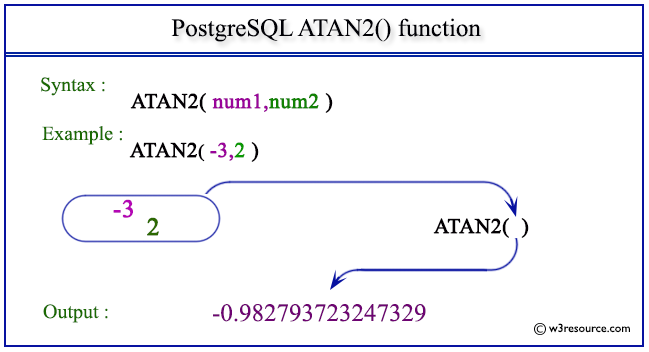# PostgreSQL ATAN2() function

## ATAN2() function

The PostgreSQL atan2() function is used to return the inverse tangent of a division given in the argument.

Syntax:

```atan2(b,a)
```

PostgreSQL Version: 9.3

Pictorial presentation of PostgreSQL ATAN2() functionExample 1: PostgreSQL ATAN2() function

Code:

``````SELECT ATAN2(0,1) AS "Atan2 (0,1)";
```
```

Sample Output:

``` Atan2 (0,1)
-------------
0
(1 row)
```

Example 2: PostgreSQL ATAN2() function

Code:

``````SELECT ATAN2(1,0) AS "Atan2 (1,0)";
```
```

Sample Output:

```   Atan2 (1,0)
-----------------
1.5707963267949
(1 row)
```

Example 3: PostgreSQL ATAN2() function

Code:

``````SELECT ATAN2(1,-1) AS "Atan2 (1,-1)";
```
```

Sample Output:

```   Atan2 (1,-1)
------------------
2.35619449019234
(1 row)
```

Example 4: PostgreSQL ATAN2() function

Code:

``````SELECT ATAN2(-3,2) AS "Atan2 (-3,2)";
```
```

Sample Output:

```    Atan2 (-3,2)
--------------------
-0.982793723247329
(1 row)
```

Previous: ATAN function
Next: CBRT function

﻿